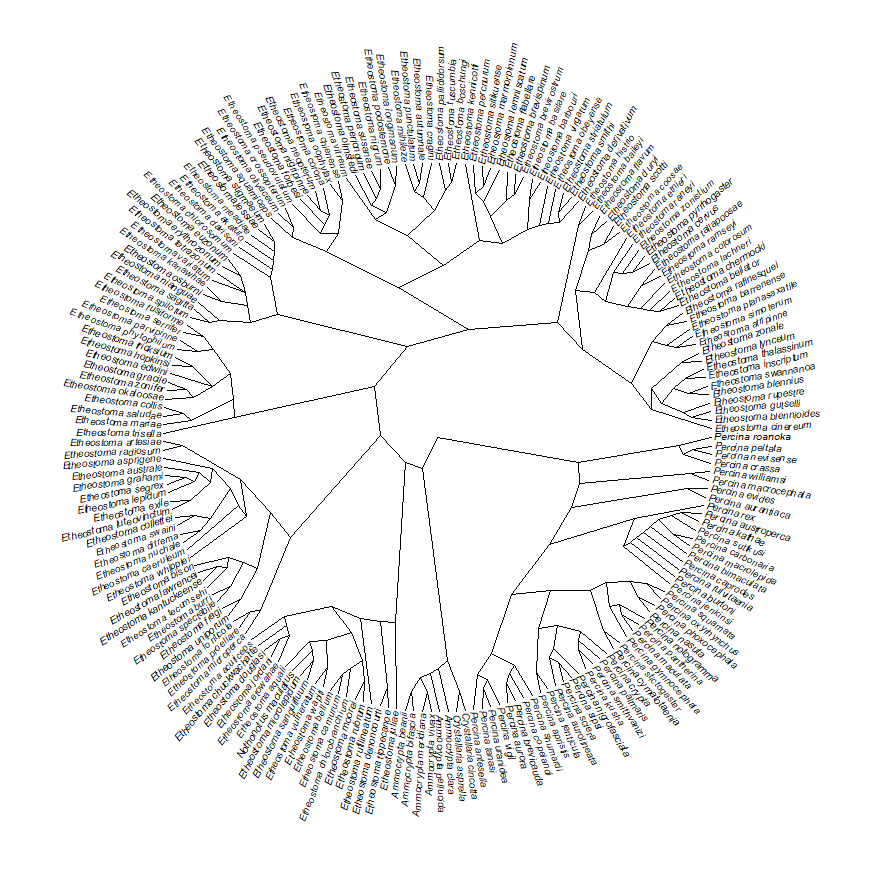## Saturday, March 28, 2020

### Major updates to mcmcMk for Bayesian MCMC with the Mk model

In the midst of all the craziness that is March of 2020, for some reason over the past few days I have been trying to improve the phytools function mcmcMk, just to see if I can possibly make it useful for some phytools users. mcmcMk does MCMC for the Mk model - but note that this can also been done using the package diversitree, and (in fact) this is what I would generally recommend.

Nonetheless, I have managed to make mcmcMk kind of cool - so it could be worth checking out.

The first thing I had to do was address some issues with the default prior distribution. I had been using an exponential distribution - but this turns out to be a really terrible idea. I have now switched to a Gamma distribution, which works much, much better.

I also (2) let the parsimony score for the trait inform the default prior. In particular, I set the default value of $$\alpha$$/$$\beta$$ (the mean from the $$\Gamma$$ distribution) to be the parsimony score divded by the total tree length. The default value of $$\alpha$$ is (for now) 0.1, which corresponds to a pretty flat distribution (though you'll see that I will use an even lower value of $$\alpha$$ here).

The other thing (3) I was able to accomplish was to speed up the likelihood calculation - which in turn speeds up the MCMC. I had been doing this using fitMk in phytools for a fixed value of the transition matrix Q. I realized that it should run faster if I just modified fitMk to export the likelihood function, and then used this likelihood function in the MCMC calculations.

In addition to this (4), I also tested geiger::fitDiscrete which already exported it's likelihood function. It turns out that fitDiscrete's likelihood calculation is significantly faster than fitMk. As such, I have now added this method as the default for computing the likelihood - but users can still use fitMk if they want. (They'll need to, for instance, if they don't have geiger installed or if they want to input their data for x as a probability matrix.)

Finally (5), I added the option (also the default) to auto-tune the proposal distribution to target a user-specified acceptance rate for the MCMC. This value defaults to 0.5, but can be adjusted up or down by the user. (For a one-parameter model, a good target acceptance rate is 0.44; for more complex models it should be lower.)

Let's test it out with some simple data:

library(phytools)
packageVersion("phytools")

##  '0.7.23'

## here's our data
tree

##
## Phylogenetic tree with 100 tips and 99 internal nodes.
##
## Tip labels:
##  t7, t44, t80, t81, t21, t18, ...
##
## Rooted; includes branch lengths.

x

##   t7  t44  t80  t81  t21  t18  t13  t84  t85  t11  t12   t2   t4  t78  t79  t27
##    0    1    1    1    1    1    0    0    0    1    1    0    1    1    1    1
##  t36  t70  t71  t42  t43  t14  t15  t73  t74  t69   t5   t1  t52  t53  t97  t98
##    1    1    1    0    1    1    1    0    1    0    0    1    0    1    1    1
##  t47  t48  t75  t76  t72  t28  t35  t37  t93  t94  t46  t99 t100  t30  t56  t90
##    1    1    1    1    1    1    0    1    0    0    0    0    0    1    0    0
##  t91  t50  t31  t23  t24  t61  t62  t67  t68  t20  t88  t89  t77  t54  t55  t39
##    1    0    1    1    0    0    1    1    1    0    0    0    0    0    1    1
##  t63  t64  t57  t58  t45   t3  t34  t86  t87  t59  t60  t51  t25  t26  t82  t83
##    1    1    1    1    0    1    1    1    1    0    0    0    1    1    1    1
##  t32  t33   t9  t10  t19  t65  t66  t29   t6   t8  t22  t49  t95  t96  t92  t16
##    0    1    1    1    0    1    1    1    0    0    0    1    1    1    1    1
##  t17  t38  t40  t41
##    1    0    1    0
## Levels: 0 1

mcmc<-mcmcMk(tree,x,ngen=2000,model="ER",prior=list(alpha=0.001))

## Running MCMC....
## gen  [1,0]   logLik  accept
## 1    0.2956  -67.298
## 101  0.757   -62.0417    0.59
## 201  0.8819  -61.9181    0.6
## 301  0.6133  -62.529 0.5
## 401  1.5944  -62.9026    0.55
## 501  1.2939  -62.3513    0.56
## 601  1.2689  -62.3083    0.54
## 701  0.766   -62.0253    0.5
## 801  0.8051  -61.9692    0.49
## 901  0.7627  -62.0312    0.5
## 1001 1.4013  -62.544 0.57
## 1101 1.091   -62.0426    0.61
## 1201 1.1005  -62.0543    0.5
## 1301 2.497   -64.3112    0.44
## 1401 1.4033  -62.5477    0.46
## 1501 0.5925  -62.6448    0.53
## 1601 0.9704  -61.9339    0.54
## 1701 0.5553  -62.8899    0.44
## 1801 0.6921  -62.2035    0.47
## 1901 1.4791  -62.6882    0.47
## Done.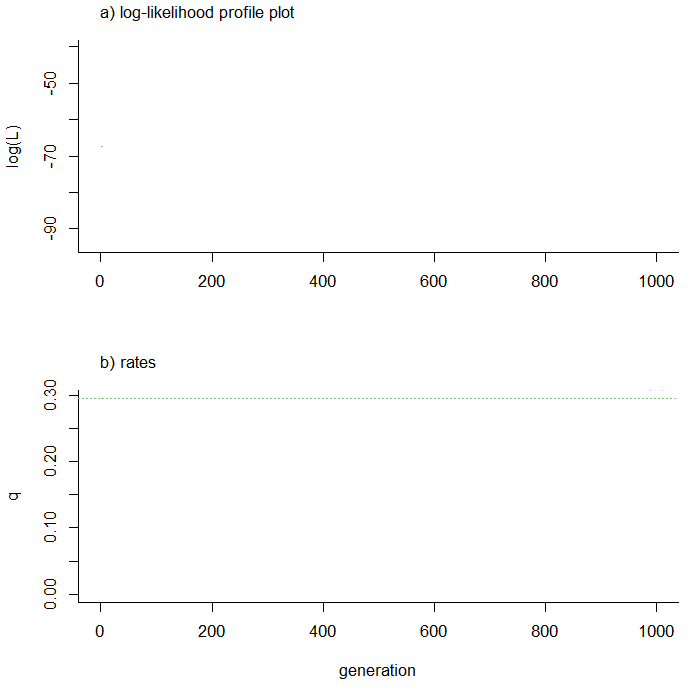Now, let's compare it to our fitted ML model:

summary(mcmc)

## Assuming 20% burn-in as no burn-in was specified....
##
## Mean value of Q from the post burn-in posterior sample:
##           0         1
## 0 -1.037971  1.037971
## 1  1.037971 -1.037971
##
## Median value of Q from the post burn-in posterior sample:
##            0          1
## 0 -0.9082315  0.9082315
## 1  0.9082315 -0.9082315
##
## 95% HPD interval computed either from the post burn-in
## samples or using 'coda':
##           lower    upper
## [1,0] 0.3608725 1.910995

fitMk(tree,x,model="ER")

## Object of class "fitMk".
##
## Fitted (or set) value of Q:
##           0         1
## 0 -0.904984  0.904984
## 1  0.904984 -0.904984
##
## Fitted (or set) value of pi:
##   0   1
## 0.5 0.5
##
## Log-likelihood: -61.915559
##
## Optimization method used was "nlminb"


Or, perhaps more meaningful, let's compare our posterior distribution on the transition rate, q, to the likelihood surface. Since our prior distribution was chosen to be quite uninformative, these should be similar:

par(mfrow=c(2,1))
plot(density(mcmc))

## Assuming 20% burn-in as no burn-in was specified....

## to compute our likelihood surface:
Mk.surf<-function(tree,x,range.q){
m<-length(unique(x))
Q<-matrix(1,m,m,dimnames=list(levels(x),levels(x)))
diag(Q)<--rowSums(Q)+1
index.matrix<-Q
diag(index.matrix)<-rep(NA,m)
lik.f<-fitMk(tree,x,model="ER")$lik q<-seq(range.q,range.q,length.out=100) logL<-sapply(q,function(q,Q) lik.f(q*Q),Q=Q) plot(q,exp(logL),type="l",bty="l", main="likelihood surface for q",font.main=1) } Mk.surf(tree,x,range(density(mcmc)$Density$x))  ## Assuming 20% burn-in as no burn-in was specified....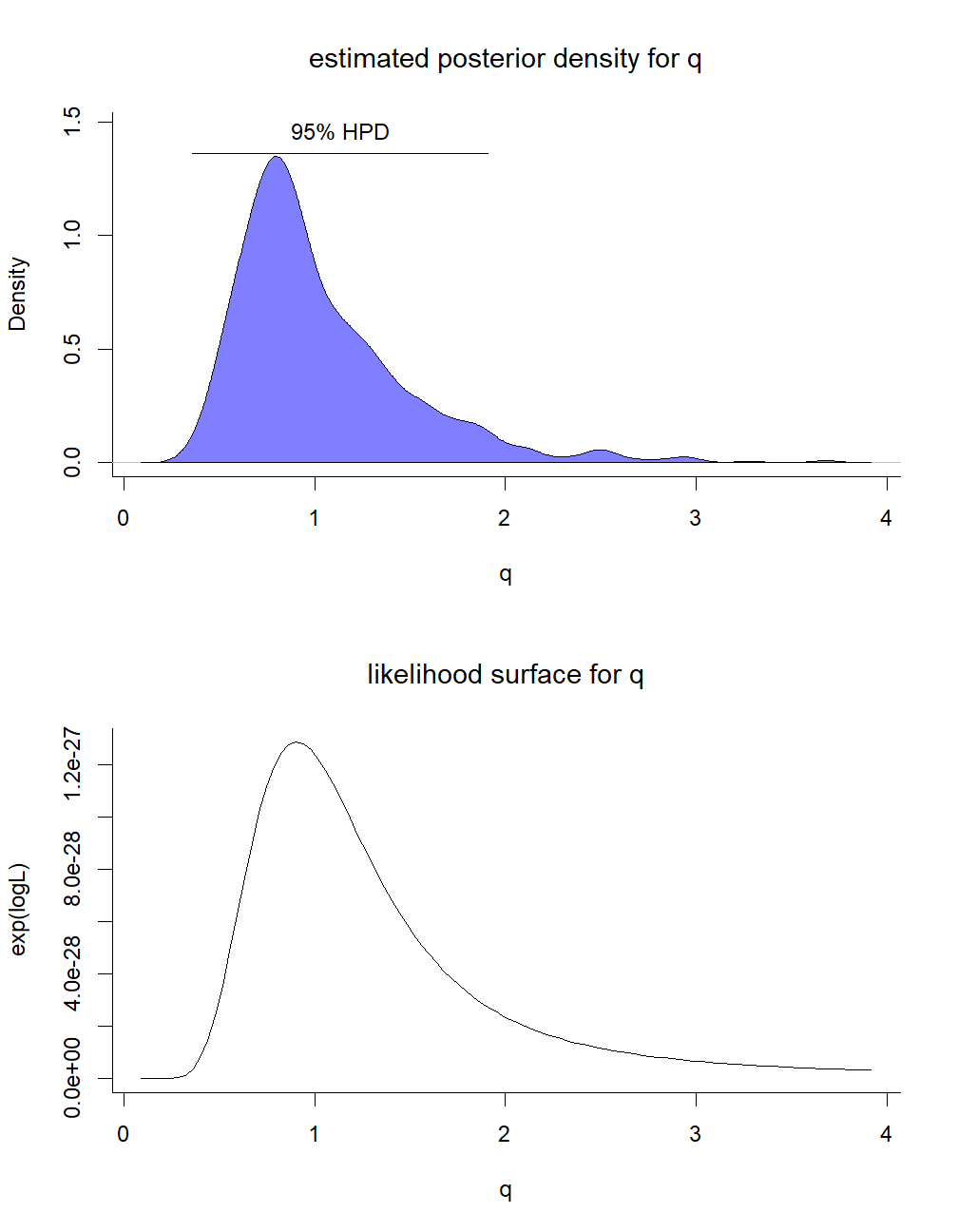That's pretty cool so far. Now, for fun, let's try an “ARD” model: mcmc<-mcmcMk(tree,x,ngen=2000,model="ARD",prior=list(alpha=0.1), auto.tune=0.3)  ## Running MCMC.... ## gen [1,0] [0,1] logLik accept ## 1 0.2956 0.2956 -67.298 ## 101 0.6481 1.3574 -59.8223 0.4 ## 201 0.636 1.5911 -60.5524 0.4 ## 301 0.6464 0.7386 -61.3897 0.11 ## 401 1.3343 3.2096 -61.7304 0.38 ## 501 1.4642 2.3867 -60.42 0.49 ## 601 1.6126 2.0809 -61.1757 0.35 ## 701 0.607 0.7238 -61.3178 0.18 ## 801 0.6791 1.2245 -59.5928 0.24 ## 901 1.6073 3.1604 -61.0521 0.25 ## 1001 0.8206 1.5465 -59.5629 0.31 ## 1101 1.3371 2.1958 -60.1967 0.24 ## 1201 1.1337 1.7848 -59.8579 0.3 ## 1301 0.8941 1.0917 -60.4888 0.26 ## 1401 1.1789 2.1283 -60.0104 0.3 ## 1501 0.6855 1.4508 -59.8168 0.26 ## 1601 1.0183 1.0874 -61.4304 0.41 ## 1701 0.6388 1.8892 -61.7907 0.23 ## 1801 0.4876 1.2122 -60.8139 0.22 ## 1901 0.589 1.1416 -59.8566 0.29 ## Done.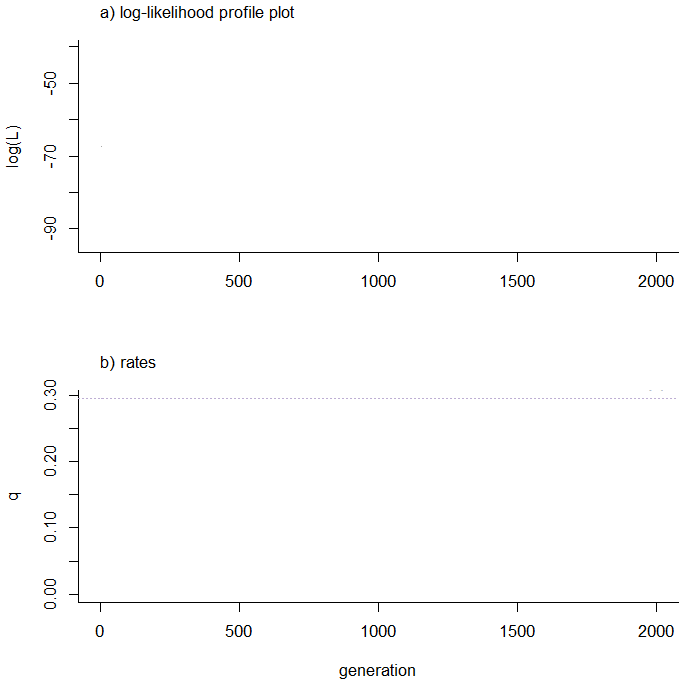(This probably needs to run longer - but you get the idea.) summary(mcmc)  ## Assuming 20% burn-in as no burn-in was specified.... ## ## Mean value of Q from the post burn-in posterior sample: ## 0 1 ## 0 -1.4867913 1.4867913 ## 1 0.8876883 -0.8876883 ## ## Median value of Q from the post burn-in posterior sample: ## 0 1 ## 0 -1.325659 1.325659 ## 1 0.777271 -0.777271 ## ## 95% HPD interval computed either from the post burn-in ## samples or using 'coda': ## lower upper ## [1,0] 0.2959638 1.805645 ## [0,1] 0.4251765 3.080158  fitMk(tree,x,model="ARD")  ## Object of class "fitMk". ## ## Fitted (or set) value of Q: ## 0 1 ## 0 -1.394135 1.394135 ## 1 0.804726 -0.804726 ## ## Fitted (or set) value of pi: ## 0 1 ## 0.5 0.5 ## ## Log-likelihood: -59.489243 ## ## Optimization method used was "nlminb"  plot(density(mcmc))  ## Assuming 20% burn-in as no burn-in was specified....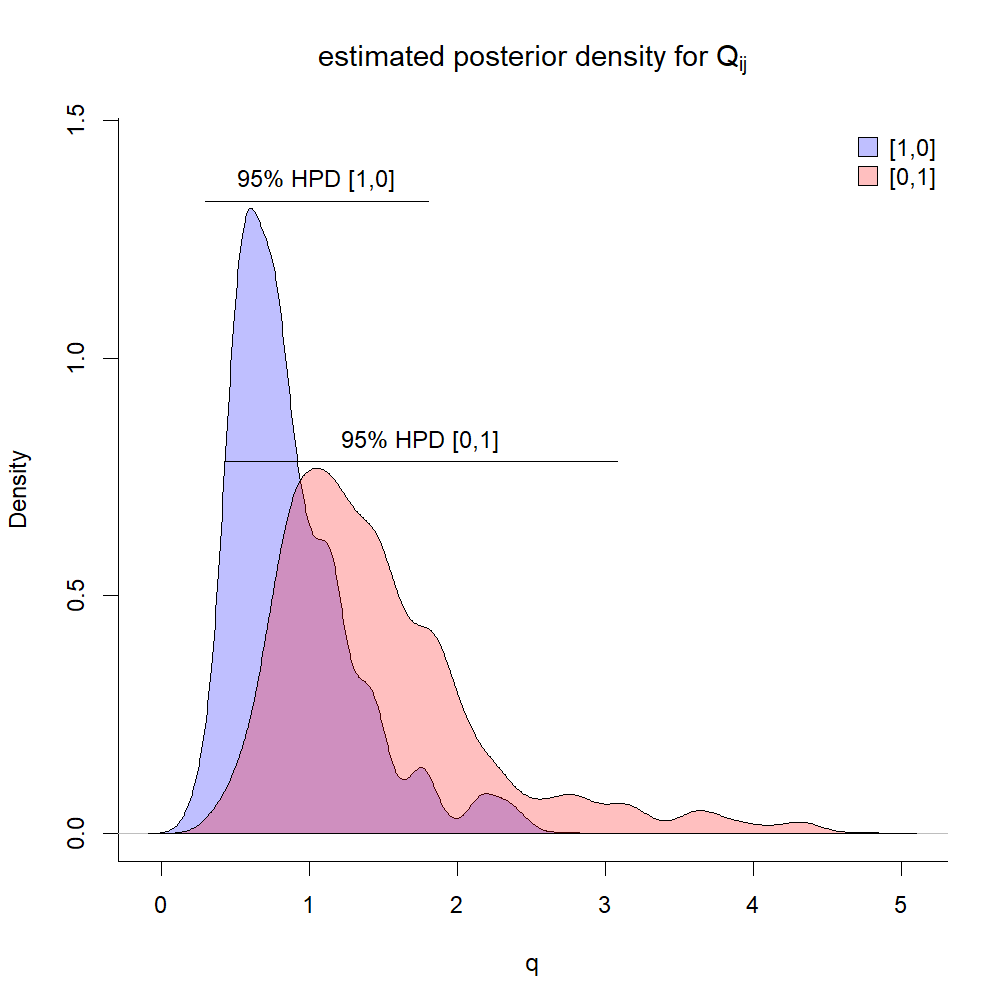That's kind of cool, right? The data for this exercise were simulated as follows: x<-sim.Mk(tree<-pbtree(n=100),Q<-matrix(c(-2,2,1,-1),2,2, dimnames=list(0:1,0:1),byrow=TRUE))  ## Sunday, January 5, 2020 ### Slanted fan-style tree using phytools Here at the SSB stand-alone meeting (aka. Systematics in the Swamp) in Gainesville, Florida I just saw a talk by Matt McGee in which he showed the following circular (aka. 'fan') slanted tree: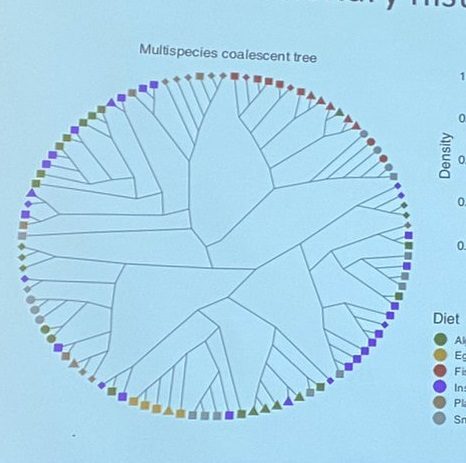So far as I know, there is no option to create such a tree in R. It's not hard to do yourself, however. Let's see how. To do it, I will using plotTree and phylomorphospace. We will use plotTree to find compute the x, y coordinates of the nodes & tips, and then we'll use phylomorphospace to draw the lines between them! library(phytools) tree<-pbtree(n=200) ## simulated tree ## preliminaries plotTree(tree,fsize=0.5,type="fan",lwd=1, ftype="off")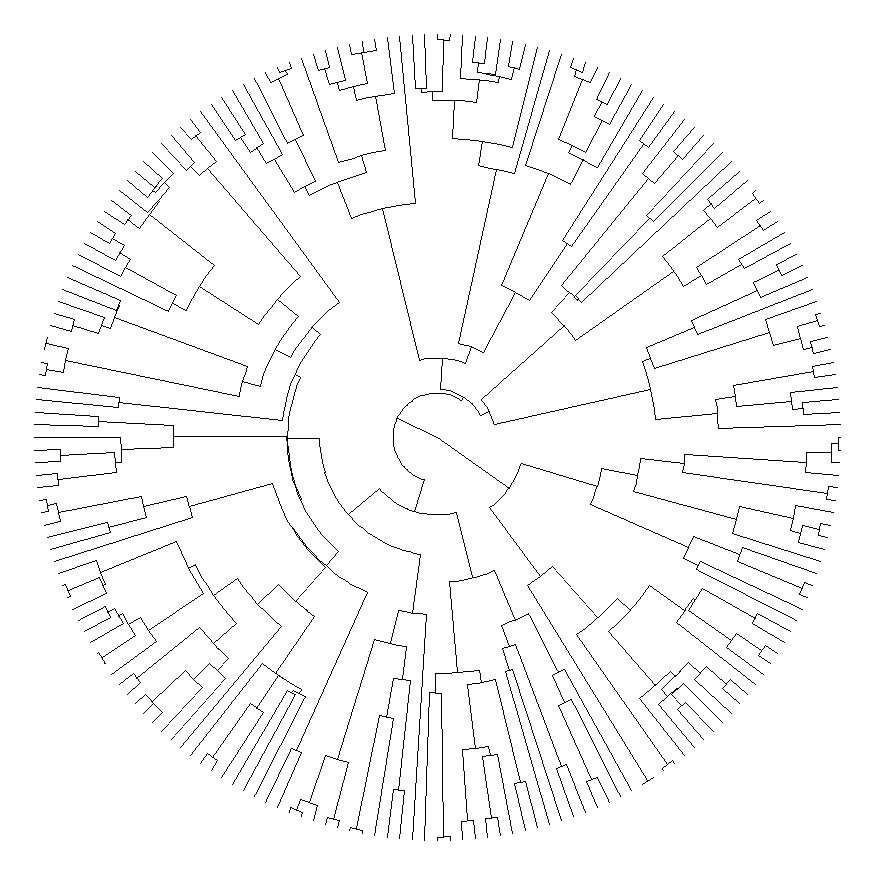obj<-get("last_plot.phylo",envir=.PlotPhyloEnv) X<-cbind(obj$xx[1:Ntip(tree)],obj$yy[1:Ntip(tree)]) rownames(X)<-tree$tip.label
A<-cbind(obj$xx[1:tree$Nnode+Ntip(tree)],
obj$yy[1:tree$Nnode+Ntip(tree)])
rownames(A)<-1:tree$Nnode+Ntip(tree) ## now let's create our plot par(fg="transparent") ## to turn off axes phylomorphospace(tree,X=X,A=A,ftype="off", node.size=c(0,0),xlim=obj$x.lim,
ylim=obj$y.lim,axes=FALSE)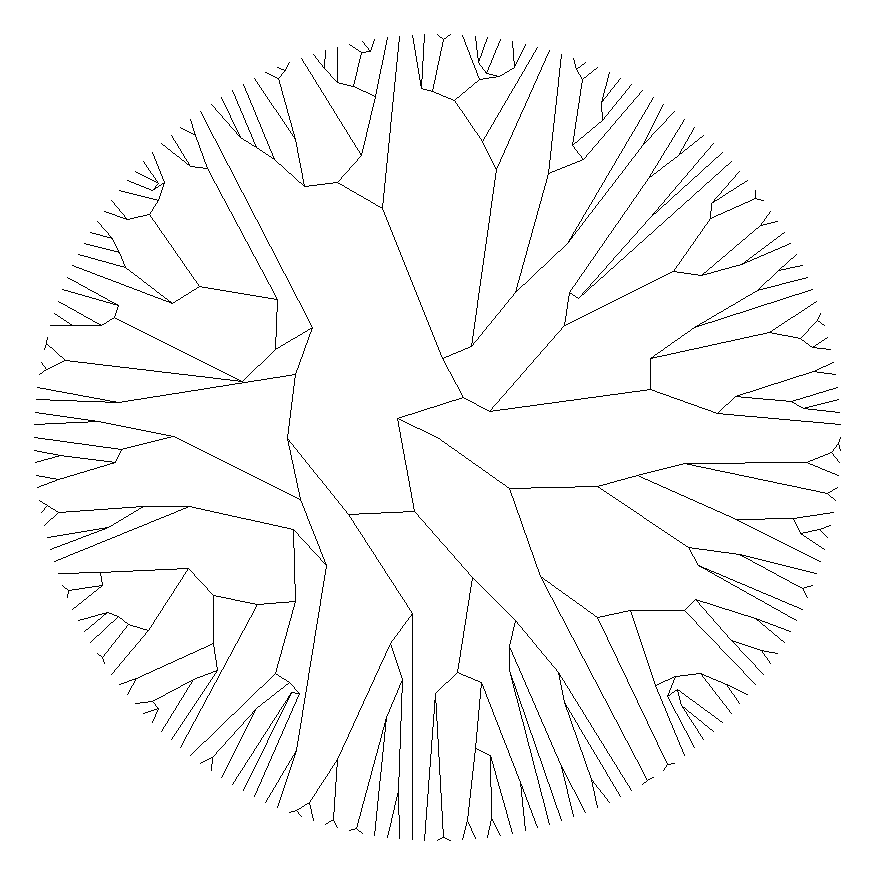par(fg="black")  If we'd like, we can also do this showing the topology only, as is often done with slanted trees. Here, I'll use an empirical tree from Near et al. (2011) that can be downloaded here. darter.tree<-read.tree(file="http://www.phytools.org/blog/darter.tre") plotTree(compute.brlen(darter.tree,power=0.5), fsize=0.5,type="fan",lwd=1,fsize=0.3, ftype="i")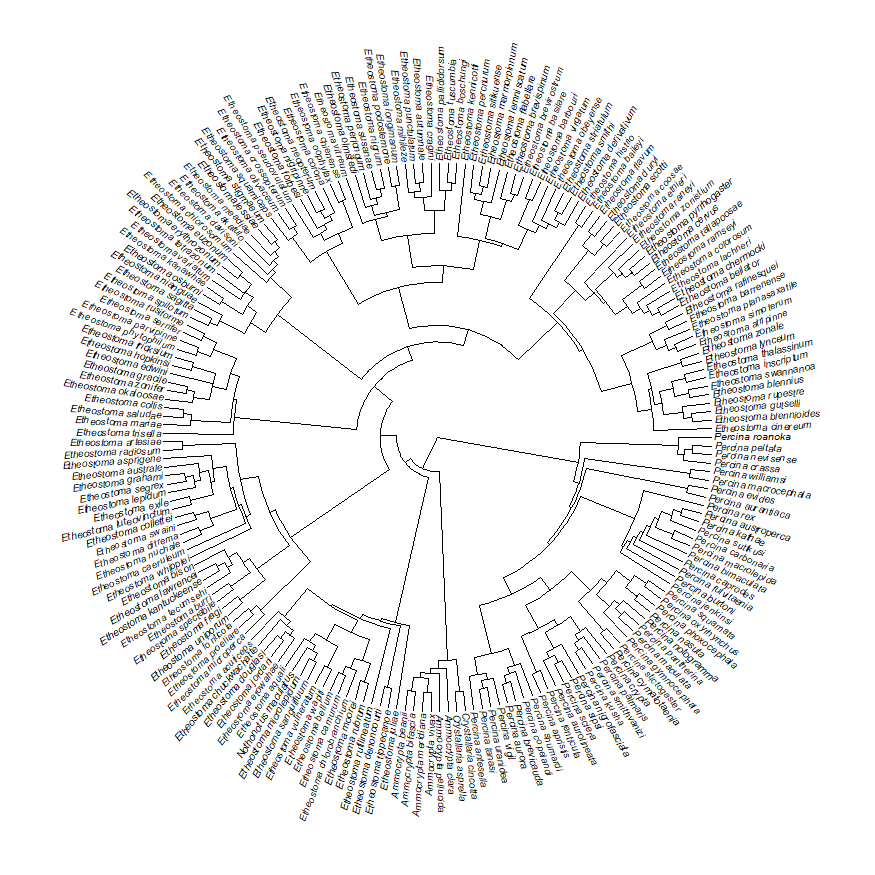obj<-get("last_plot.phylo",envir=.PlotPhyloEnv) X<-cbind(obj$xx[1:Ntip(darter.tree)],
obj$yy[1:Ntip(darter.tree)]) rownames(X)<-darter.tree$tip.label
A<-cbind(obj$xx[1:darter.tree$Nnode+
Ntip(darter.tree)],
obj$yy[1:darter.tree$Nnode+
Ntip(darter.tree)])
rownames(A)<-1:darter.tree$Nnode+ Ntip(darter.tree) par(fg="transparent") phylomorphospace(darter.tree,X=X,A=A,ftype="off", node.size=c(0,0),xlim=obj$x.lim,
ylim=obj\$y.lim,xaxt="n")
par(fg="black")
## as a last step we can put our labels back on
plotTree(darter.tree,fsize=0.5,type="fan",lwd=1,